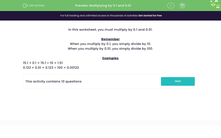# Multiply by 0.1 and 0.01

In this worksheet, students will practise multiplying by 0.1 and 0.01.Key stage:  KS 3

Curriculum topic:   Number

Curriculum subtopic:   Use Four Operations for All Numbers

Difficulty level:#### Worksheet Overview

In this activity, we will multiply numbers by 0.1 and 0.01.

When you multiply by 0.1, you are simply dividing by 10.

When you multiply by 0.01, you are simply dividing by 100.

Examples

15.1 × 0.1 = 15.1 ÷ 10 = 1.51

0.123 × 0.01 = 0.123 ÷ 100 = 0.00123

When multiplying with decimal numbers, we need to count how many decimal places we have in our calculation and make sure that we have the same number in our answer!

In the first example above, we have two decimal places in the calculation: 15.1 x 0.1, so our answer must have two decimal places: 1.51

In the second example, we have five decimal places in the calculation: 0.123 x 0.01, so our answer must have five decimal places: 0.00123

If you remember this rule about decimal places, you'll find multiplying decimals becomes really simple.

Let's have a go at some questions now.

### What is EdPlace?

We're your National Curriculum aligned online education content provider helping each child succeed in English, maths and science from year 1 to GCSE. With an EdPlace account you’ll be able to track and measure progress, helping each child achieve their best. We build confidence and attainment by personalising each child’s learning at a level that suits them.

Get started